## Thue-Morse Sequence

The Integer Sequence (also called the Morse-Thue Sequence)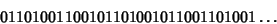(1)

(Sloane's A010060) which arises in the Thue-Morse Constant. It can be generated from the Substitution Map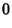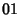(2)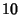(3)

starting with 0 as follows:(4)

Writing the sequence as a Power Series over the Galois Field GF(2),(5)

thensatisfies the quadratic equation(6)

This equation has two solutions,and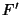, whereis the complement of, i.e.,(7)

which is consistent with the formula for the sum of the roots of a quadratic. The equality (6) can be demonstrated as follows. Let (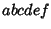...) be a shorthand for the Power series(8)

so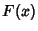is (0110100110010110...). To get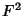, simply use the rule for squaring Power Series over GF(2)(9)

which extends to the simple rule for squaring a Power Series(10)

i.e., space the series out by a factor of 2, (0 1 1 0 1 0 0 1 ...), and insert zeros in the Odd places to get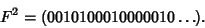(11)

Then multiply by(which just adds a zero at the front) to get(12)

Adding togives(13)

This is the first term of the quadratic equation, which is the Thue-Morse sequence with each term doubled up. The next term is, so we have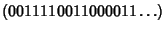(14)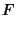(15)

The sum is the above two sequences XORed together (there are no Carries because we're working over GF(2)), giving(16)

We therefore have(17)

The Thue-Morse sequence is an example of a cube-free sequence on two symbols (Morse and Hedlund 1944), i.e., it contains no substrings of the form, where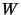is any Word. For example, it does not contain the Words 000, 010101 or 010010010. In fact, the following stronger statement is true: the Thue-Morse sequence does not contain any substrings of the form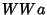, whereis the first symbol of. We can obtain a Squarefree sequence on three symbols by doing the following: take the Thue-Morse sequence 0110100110010110... and look at the sequence of Words of length 2 that appear: 01 11 10 01 10 00 01 11 10 .... Replace 01 by 0, 10 by 1, 00 by 2 and 11 by 2 to get the following: 021012021.... Then this Sequence is Squarefree (Morse and Hedlund 1944).

The Thue-Morse sequence has important connections with the Gray Code. Kindermann generates fractal music using the Self-Similarity of the Thue-Morse sequence.

References

Kindermann, L. MusiNum--The Music in the Numbers.'' http://www.forwiss.uni-erlangen.de/~kinderma/musinum/.

Morse, M. and Hedlund, G. A. Unending Chess, Symbolic Dynamics, and a Problem in Semigroups.'' Duke Math. J. 11, 1-7, 1944.

Schroeder, M. R. Fractals, Chaos, and Power Laws: Minutes from an Infinite Paradise. New York: W. H. Freeman, 1991.

Sloane, N. J. A. Sequence A010060 in The On-Line Version of the Encyclopedia of Integer Sequences.'' http://www.research.att.com/~njas/sequences/eisonline.html.